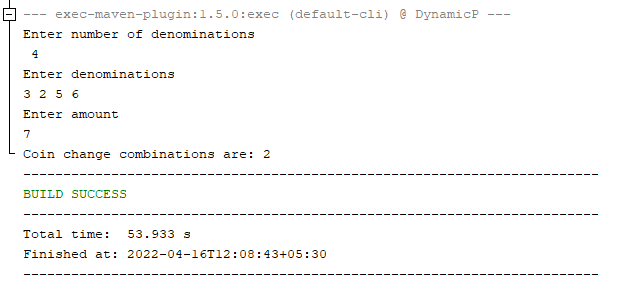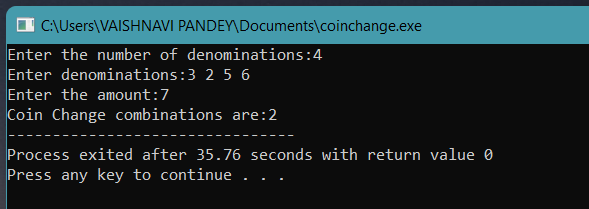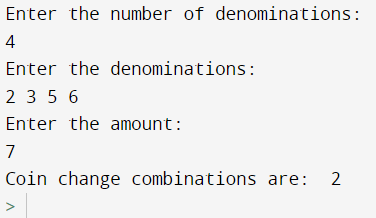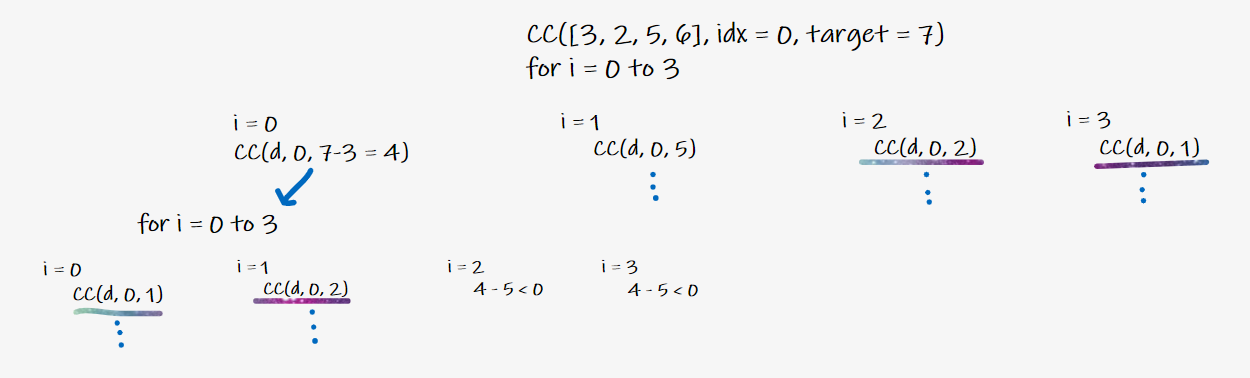# Coin Change Combination Problem

## Introduction

Today, let’s learn about a famous and commonly asked Interview Problem, i.e., Coin Change Combination Problem. It is a famous and common problem that is solved using Dynamic Programming in its most optimized version.

## Problem Statement

Given denominations of ‘N’ coins and an amount, we need to calculate the maximum number of ways(or combinations) the given amount can be paid. We are also given an infinite supply of each coin.

Example:

Denominations of a coin = [2, 3, 5, 6] and amount = 7

So, here the possible combinations are 2 + 2 + 3 = 7 (amount) and 2 + 5 = 7 (amount).

Note: We only need to consider combinations and not the permutations i.e. other valid combinations are 2 + 3 + 2 = 7, 3 + 2 + 2 = 7. But these are permutations and not combinations.

In permutations, we can consider numbers in the array in any order but while forming a combination, numbers could be considered only in forward order, i.e., after 3, an infinite supply of only Rs. 3, 5, 6 can be considered, and Rs 2 coin cannot be considered.

Let's get started and learn various approaches to solve this problem.

Recommended: Try the Problem yourself before moving on to the solution

## Approach 1: Brute Force(Using Recursion)

The naive (or brute force) solution to this problem could be finding all possible configurations of different coins in denominations and finding the maximum ways possible for the Coin Change Combination Problem. To know more about recursion follow this link.

The base case would be that if the amount is 0, the only possible way would be 1, i.e., not selecting any coin. If the denomination array is empty and the amount is non-zero, then we can never form the given amount; hence the max number of ways for this would be 0. For all other cases, every coin in the denomination array would have 2 choices, i.e., to get selected or not.

### Implementation in Java

Below is the implementation of this problem in various languages.

``````import java.io.*;
import java.util.*;

public class Main {

public static void main(String[] args) throws Exception {
Scanner s = new Scanner(System.in);

System.out.println("Enter number of denominations");
int n = s.nextInt();
int[] denominations = new int[n];

System.out.println("Enter denominations");
for (int i = 0; i < n; i++) denominations[i] = s.nextInt();

System.out.println("Enter amount");
int target = s.nextInt();

int ways = coinCombinations(denominations, 0, target);
System.out.print("Coin change combinations are: ");
System.out.println(ways);
}

// Recursive approach
public static int coinCombinations(int[] denominations, int idx, int target){

// Base Case
if (target == 0) return 1;

int ways = 0;
for (int i = idx; i < denominations.length; i++){

// Counting ways if i-th denomination cam be included
if (target - denominations[i] >=0 )
ways += coinCombinations(denominations, i, target-denominations[i]);
}
return ways;
}
}``````

Output### Implementation in C++

``````#include <bits/stdc++.h>

using namespace std;

int coinChangeHelper(vector < int > & coins, int start, int end, int amount) {
if (start > end) {
if (amount == 0) {
return 0;
}
return -1;
}
// If amount is negative
if (amount < 0) {
return -1;
}

// Recursive calls
int ans1 = coinChangeHelper(coins, start, end, amount - coins[start]);
int ans2 = coinChangeHelper(coins, start + 1, end, amount);

if (ans1 != -1 && ans2 != -1) {
return min(ans1 + 1, ans2);
}

// If we cannot achieve that amount through recursive call 1
if (ans1 == -1) {
return ans2;
}

// If we cannot achieve that amount through recursive call 2
if (ans2 == -1) {
return (ans1 + 1);
}

return -1;
}

int coinChange(vector < int > & coins, int amount) {
return coinChangeHelper(coins, 0, coins.size() - 1, amount);
}

int main() {

cout << "Enter the number of denominations:";
int n;
cin >> n;

cout << "Enter denominations:";
vector < int > coins;
for (int i = 0; i < n; i++) {
int elem;
cin >> elem;
coins.push_back(elem);
}

cout << "Enter the amount:";
int amount;
cin >> amount;

// Calling the function
cout << "Coin Change combinations are:";
cout << coinChange(coins, amount);
return 0;
}``````

Output### Implementation in Python

``````def main():

# No of denominations
print('Enter the number of denominations:')
n = int(input())
# denominations
print('Enter the denominations:')
denominations = list(map(int, input().split()))
# amount
print('Enter the amount:')
target = int(input())

# Recursive approach
def coinCombinations(denominations, idx, target):

# Base Case
if target == 0: return 1

ways = 0
for i in range(idx, len(denominations)):

# Counting ways if i-th denomination cam be included
if target - denominations[i] >=0:
ways += coinCombinations(denominations, i, target-denominations[i])

return ways
print('Coin change combinations are:')
res = coinCombinations(denominations, 0, target)
print("Total combinations are", res)

main()``````

Output### Complexity Analysis

• Time Complexity: The time complexity is O(N ^ amount) as for each denomination, we calculate the number of ways to form all the amounts (i.e. from 1 to a given amount).
• Space Complexity: O(1) as no extra space is being used. Where ‘N’ is the number of denominations.

## Approach 2: Using Dynamic Programming

We could optimize the time complexity of our previous approach by maintaining a DP array where DP [i] stores the max possible ways for each amount (i.e. 1 to a given amount).

Let's look at the recursive tree:Since the recursive approach had a lot of overlapping subproblems, the DP array would also help us avoid that repetitive work.

### Approach 2a: Using Top-Down DP

Below is the implementation of this problem in various languages.

#### Implementation in Java

``````import java.io.*;
import java.util.*;

public class Main {

public static void main(String[] args) throws Exception {
Scanner s = new Scanner(System.in);

System.out.println("Enter number of denominations");
int n = s.nextInt();
int[] denominations = new int[n];

System.out.println("Enter denominations");
for (int i = 0; i < n; i++)
{
denominations[i] = s.nextInt();
}

System.out.println("Enter amount");
int target = s.nextInt();

// Forming Dp Array
int[][] dp = new int[denominations.length][target + 1];

int ways = coinCombinations(denominations, 0, target, dp);

System.out.print("Coin change combinations are: ");
System.out.println(ways);
}

// Top Down DP approach
public static int coinCombinations(int[] denominations, int idx, int target, int[][] dp){

// Base Case
if (target == 0) return 1;

// LookUp in Dp Array
if (dp[idx][target] != 0) return dp[idx][target];

int ways = 0;
for (int i = idx; i < denominations.length; i++){

// Counting ways if i-th denomination can be included
if (target - denominations[i] >=0 )
ways += coinCombinations(denominations, i, target-denominations[i], dp);
}
return dp[idx][target] = ways;
}
}``````

Output#### Implementation in C++

``````#include <bits/stdc++.h>

using namespace std;

int coinChangeHelper(vector < int > & coins, int start, int end, int amount, int ** mem) {

// Base Case
if (start > end) {
if (amount == 0) {
return 0;
}
return -1;
}

// If amount is negative
if (amount < 0) {
return -1;
}

// If result of that recursive call already present
if (mem[start][amount] != -1) {
return mem[start][amount];
}

// Recursive calls
int ans1 = coinChangeHelper(coins, start, end, amount - coins[start], mem);
int ans2 = coinChangeHelper(coins, start + 1, end, amount, mem);

if (ans1 != -1 && ans2 != -1) {
mem[start][amount] = min(ans1 + 1, ans2);
return mem[start][amount];
}
if (ans1 == -1) {
mem[start][amount] = ans2;
return mem[start][amount];
}
if (ans2 == -1) {
mem[start][amount] = (ans1 + 1);
return mem[start][amount];
}

return -1;
}

int coinChange(vector < int > & coins, int amount) {

// Forming the array for storing the results of the recursive calls
int ** mem = new int * [coins.size()];
for (int i = 0; i < coins.size(); i++) {
mem[i] = new int[amount + 1];
for (int j = 0; j <= amount; j++) {
mem[i][j] = -1;
}
}
return coinChangeHelper(coins, 0, coins.size() - 1, amount, mem);
}

int main() {

cout << "Enter the number of denominations:";
int n;
cin >> n;

cout << "Enter denominations:";
vector < int > coins;
for (int i = 0; i < n; i++) {
int elem;
cin >> elem;
coins.push_back(elem);
}

cout << "Enter the amount:";
int amount;
cin >> amount;

// Calling the function
cout << "Coin Change combinations are:";
cout << coinChange(coins, amount);
return 0;
}``````

Output#### Implementation in Python

``````def main():

# No of denominations
print('Enter the number of denominations:')
n = int(input())
print('Enter the denominations:')
# denominations
denominations = list(map(int, input().split()))
# amount
print('Enter the amount:')
target = int(input())

# Top Down DP approach
def coinCombinations(denominations, idx, target, dp):

# Base Case
if target == 0: return 1

# LookUp in Dp Array
if dp[idx][target] != 0: return dp[idx][target]

ways = 0
for i in range(idx, len(denominations)):

# Counting ways if i-th denomination can be included
if target - denominations[i] >=0:
ways += coinCombinations(denominations, i, target-denominations[i], dp);

dp[idx][target] = ways
return ways

# Forming Dp Array
dp = [[0 for j in range(target+1)] for i in range(len(denominations))]
ways = coinCombinations(denominations, 0, target, dp)

print("Coin change combinations are: ", ways)
main()``````

Output#### Complexity Analysis

• Time Complexity: O(N * amount) as for each denomination, we calculate the number of ways to form all the amounts (i.e. from 1 to a given amount).
• Space Complexity: O(amount) as extra space for storing the max possible ways for each amount that has been used.

Where ‘N’ is the number of denominations.

### APPROACH 2b: Using Bottom-Up Dp

Below is the implementation of this problem in various languages.

#### Implementation in Java

``````import java.io.*;
import java.util.*;

public class CoinChange {

public static void main(String[] args) throws Exception {
Scanner s = new Scanner(System.in);

System.out.println("Enter number of denominations");
int n = s.nextInt();
int[] denominations = new int[n];

System.out.println("Enter denominations");
for (int i = 0; i < n; i++) denominations[i] = s.nextInt();

System.out.println("Enter amount");
int target = s.nextInt();

coinCombinationsDp(denominations, target);

}

// Bottom Up DP approach
public static void coinCombinationsDp(int[] denominations, int target) {

// Forming dp array
int[] dp = new int[target + 1];

/*
precompute
0 amount could be get in 1 way (i.e. not selecting any coin)

*/
dp = 1;

// Counting coin change combinations
for (int i = 0; i < denominations.length; i++) {
for (int j = 1; j < dp.length; j++) {
if (j - denominations[i] < 0) continue;
dp[j] += dp[j - denominations[i]];
}
}

System.out.print("Coin change combinations are: ");
System.out.println(dp[dp.length - 1]);
}

}``````

Output#### Implementation in C++

``````#include <bits/stdc++.h>

using namespace std;

int coinChange(vector < int > & coins, int amount) {
int n = coins.size();

// 2-D array for storing the results
int ** dp = new int * [n + 1];
for (int i = 0; i <= n; i++) {
dp[i] = new int[amount + 1];
}

// Traversing the 2-D dp array
for (int i = 0; i <= n; i++) {
for (int j = 0; j <= amount; j++) {

// If size of the array is zero and amount is also zero
if (i == 0 && j == 0) {
dp[i][j] = 0;
}

// If size of the array is zero
else if (i == 0) {
dp[i][j] = -1;
}

// If the amount is zero
else if (j == 0) {
dp[i][j] = 0;
} else {

// If the value of current coin is less than the amount
if (j - coins[i - 1] >= 0) {
if (dp[i][j - coins[i - 1]] != -1 && dp[i - 1][j] != -1)
dp[i][j] = min(dp[i][j - coins[i - 1]] + 1, dp[i - 1][j]);

// If we can't take the current coin for making our amount
else if (dp[i][j - coins[i - 1]] == -1) {
dp[i][j] = dp[i - 1][j];
} else if (dp[i - 1][j] == -1) {
dp[i][j] = (dp[i][j - coins[i - 1]] + 1);
}
} else {
dp[i][j] = dp[i - 1][j];
}

}
}
}

return dp[n][amount];
}

int main()
{

cout << "Enter the number of denominations:";
int n;
cin >> n;

cout << "Enter denominations:";
vector < int > coins;
for (int i = 0; i < n; i++)
{
int elem;
cin >> elem;
coins.push_back(elem);
}

cout << "Enter the amount:";
int amount;
cin >> amount;

// Calling the function
cout << "Coin Change combinations are:";
cout << coinChange(coins, amount);
return 0;
}``````

Output#### Implementation in Python

``````def main():

# No of denominations
print('Enter the number of denominations:')
n = int(input())
print('Enter the denominations:')
# denominations
denominations = list(map(int, input().split()))
# amount
print('Enter the amount:')
target = int(input())

# Bottom Up DP approach
def coinCombinationsDp(denominations, target):

# Forming dp array
dp = [0 for i in range(target + 1)]

# 0 amount could be get in 1 way (i.e. not selecting any coin)
dp = 1;

# Counting coin change combinations
for i in range(len(denominations)):
for j in range(1, len(dp)):
if j - denominations[i] < 0: continue
dp[j] += dp[j- denominations[i]]

print("Coin change combinations are: ", dp[len(dp) - 1])

coinCombinationsDp(denominations, target)

main()``````

Output#### Complexity Analysis

• Time Complexity: O(N * amount) as for each denomination, we calculate the number of ways to form all the amounts (i.e. from 1 to a given amount).
• Space Complexity: O(amount) as extra space for storing the max possible ways for each amount that has been used.

Where ‘N’ is the number of denominations.

### What is dynamic programming?

Dynamic programming is both a mathematical optimization method and a computer programming method mainly used to optimize plain recursion.

### What are two ways to apply dynamic programming?

The two ways to apply DP are bottom-up (i.e., iterative way) and top-down (i.e., using dp in recursion, commonly known as memorization).

### What is the combination, and how is it different from permutation?

In permutations, we can consider numbers in the array in any order, but numbers could be considered only in forward order while forming a combination.

### Why are we optimizing using DP?

This is because the recursive approach had a lot of overlapping subproblems; the DP array would also help us avoid that repetitive work.

## Conclusion

In this blog, we learned various approaches to the Coin Change Combination.

• Coin Change Combination is a standard recursive problem that is optimized via Dynamic Programming.
• In permutations, we can consider numbers in the array in any order, but while forming a combination, numbers could be considered only in forward order.

The optimized time complexity of this problem is O(n^amount) as for each denomination, we calculate the number of ways to form all the amounts (i.e. from 1 to a given amount).

#### Recommended Problems

Practice a variety of Problems and also check out some Guided Paths on topics such as Data Structure and Algorithms and Competitive Programming, along with some Interview Bundles and Interview Experiences only on CodeStudio.

Do upvote our blog to help other ninjas grow.

Happy Learning!